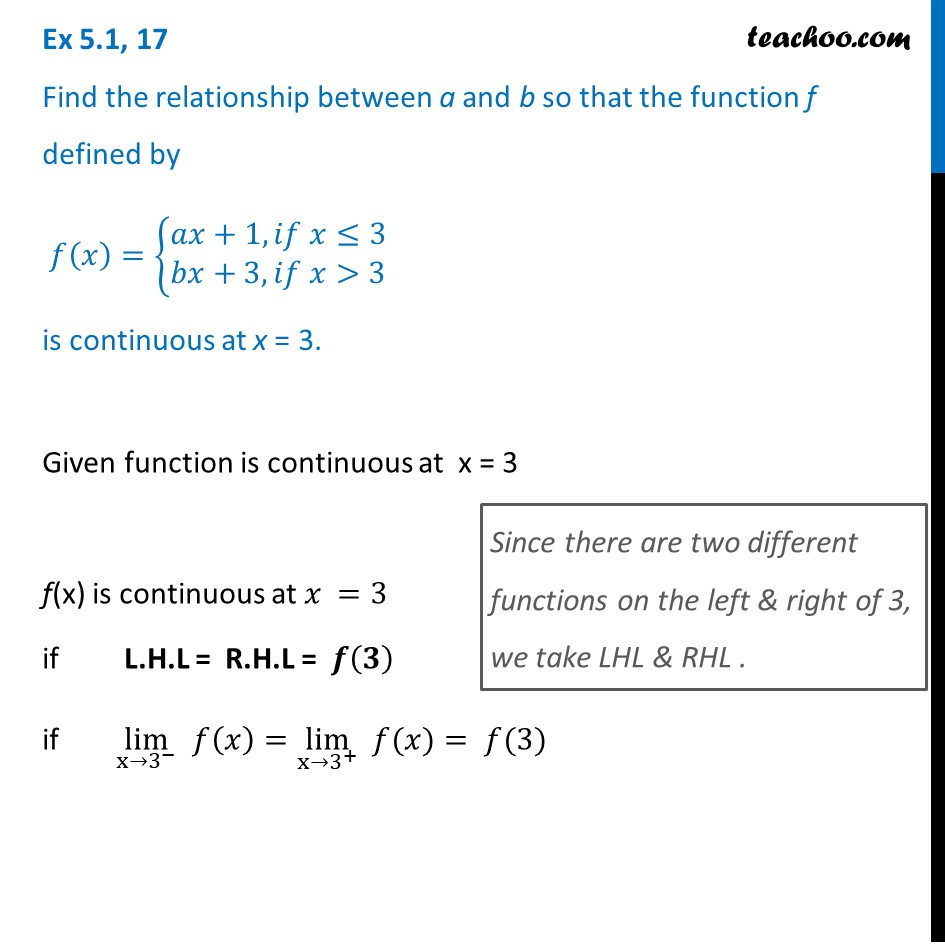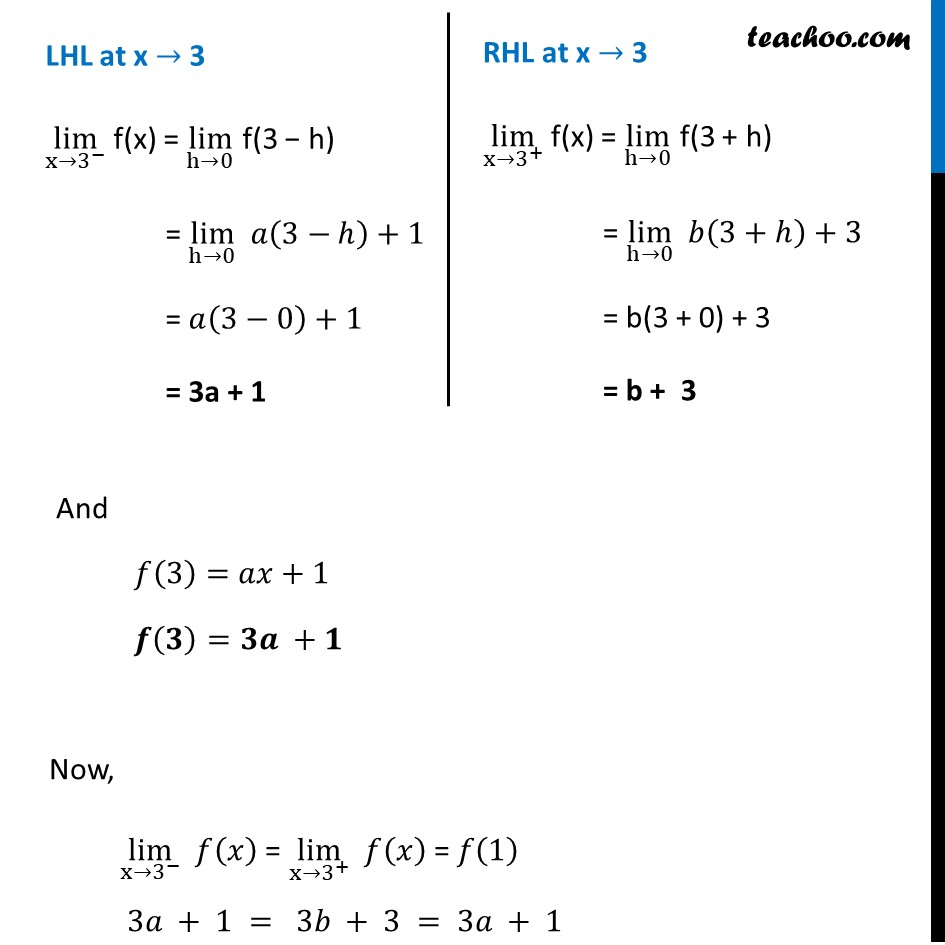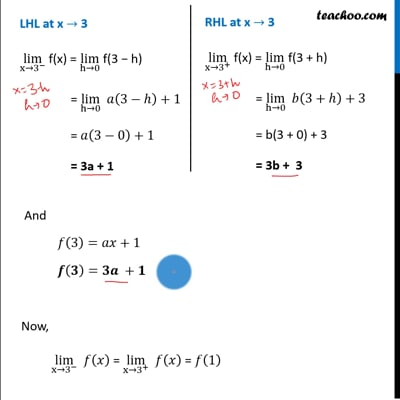Ex 5.1

Chapter 5 Class 12 Continuity and Differentiability
Serial order wiseThis video is only available for Teachoo black users

Introducing your new favourite teacher - Teachoo Black, at only ₹83 per month

### Transcript

Ex 5.1, 17 Find the relationship between a and b so that the function f defined by 𝑓(𝑥)={█(𝑎𝑥+1, 𝑖𝑓 𝑥≤3@&𝑏𝑥+3, 𝑖𝑓 𝑥>3)┤ is continuous at x = 3.Given function is continuous at x = 3 f(x) is continuous at 𝑥 =3 if L.H.L = R.H.L = 𝒇(𝟑) if lim┬(x→3^− ) 𝑓(𝑥)=lim┬(x→3^+ ) " " 𝑓(𝑥)= 𝑓(3) Since there are two different functions on the left & right of 3, we take LHL & RHL . LHL at x → 3 lim┬(x→3^− ) f(x) = lim┬(h→0) f(3 − h) = lim┬(h→0) 𝑎(3−ℎ)+1 = 𝑎(3−0)+1 = 3a + 1 RHL at x → 3 lim┬(x→3^+ ) f(x) = lim┬(h→0) f(3 + h) = lim┬(h→0) 𝑏(3+ℎ)+3 = b(3 + 0) + 3 = b + 3 And 𝑓(3)=𝑎𝑥+1 𝒇(𝟑)=𝟑𝒂 +𝟏 Now, lim┬(x→3^− ) 𝑓(𝑥) = lim┬(x→3^+ ) 𝑓(𝑥) = 𝑓(1) 3𝑎 + 1 = 3𝑏 + 3 = 3𝑎 + 1 Comparing values 𝟑𝒂 + 𝟏 = 𝟑𝒃 + 𝟑 3𝑎−3b=3−1 3𝑎 −3𝑏=2 3(𝑎−𝑏)=2 𝑎−𝑏=2/3 𝒂=𝒃+ 𝟐/𝟑 Thus , for any value of b, We can find value of a